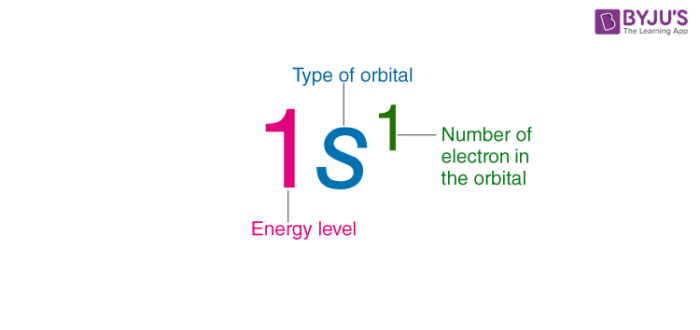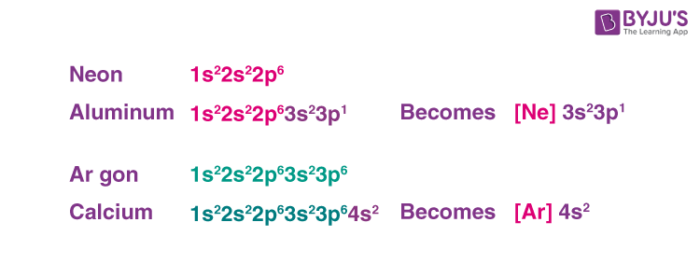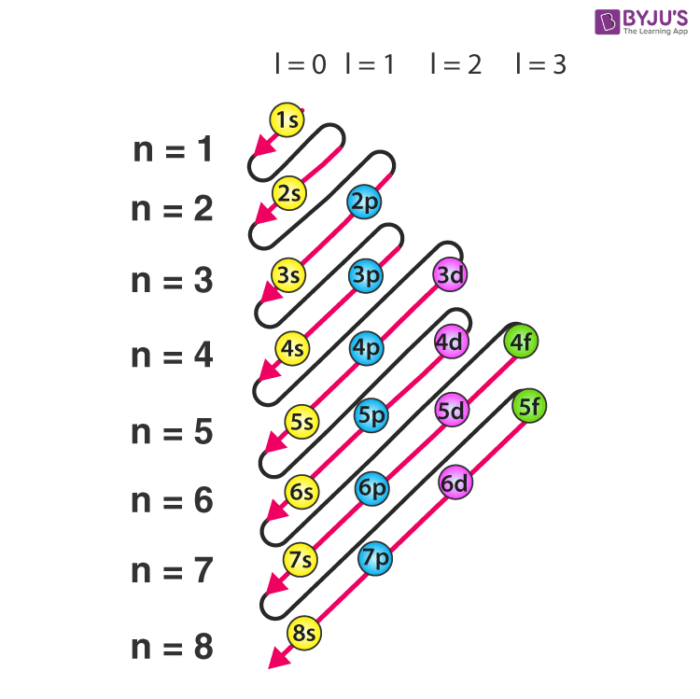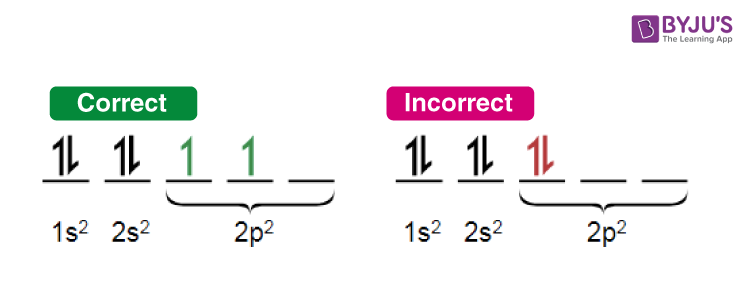Checkout JEE MAINS 2022 Question Paper Analysis : Checkout JEE MAINS 2022 Question Paper Analysis :

# Electron Configuration

## What are Electron Configurations?

The electron configuration of an element describes how electrons are distributed in its atomic orbitals. Electron configurations of atoms follow a standard notation in which all electron-containing atomic subshells (with the number of electrons they hold written in superscript) are placed in a sequence. For example, the electron configuration of sodium is 1s22s22p63s1.

## Table of ContentHowever, the standard notation often yields lengthy electron configurations (especially for elements having a relatively large atomic number). In such cases, an abbreviated or condensed notation may be used instead of the standard notation. In the abbreviated notation, the sequence of completely filled subshells that correspond to the electronic configuration of a noble gas is replaced with the symbol of that noble gas in square brackets. Therefore, the abbreviated electron configuration of sodium is [Ne]3s1 (the electron configuration of neon is 1s22s22p6, which can be abbreviated to [He]2s22p6).Electron Configurations are useful for:

• Determining the valency of an element.
• Predicting the properties of a group of elements (elements with similar electron configurations tend to exhibit similar properties).
• Interpreting atomic spectra.

This notation for the distribution of electrons in the atomic orbitals of atoms came into practice shortly after the Bohr model of the atom was presented by Ernest Rutherford and Niels Bohr in the year 1913.

## Writing Electron Configurations

### Shells

The maximum number of electrons that can be accommodated in a shell is based on the principal quantum number (n). It is represented by the formula 2n2, where ‘n’ is the shell number. The shells, values of n, and the total number of electrons that can be accommodated are tabulated below.

 Shell and ‘n’ value Maximum electrons present in the shell K shell, n=1 2*12 = 2 L shell, n=2 2*22 = 8 M shell, n=3 2*32 = 18 N shell, n=4 2*42 = 32

### Subshells

• The subshells into which electrons are distributed are based on the azimuthal quantum number (denoted by ‘l’).
• This quantum number is dependent on the value of the principal quantum number, n. Therefore, when n has a value of 4, four different subshells are possible.
• When n=4. The subshells correspond to l=0, l=1, l=2, and l=3 and are named the s, p, d, and f subshells, respectively.
• The maximum number of electrons that can be accommodated by a subshell is given by the formula 2*(2l + 1).
• Therefore, the s, p, d, and f subshells can accommodate a maximum of 2, 6, 10, and 14 electrons, respectively.

All the possible subshells for values of n up to 4 are tabulated below.

 Principle Quantum Number Value Value of Azimuthal Quantum Number Resulting Subshell in the Electron Configuration n=1 l=0 1s n=2 l=0 2s l=1 2p n=3 l=0 3s l=1 3p l=2 3d n=4 l=0 4s l=1 4p l=2 4d l=3 4f

Thus, it can be understood that the 1p, 2d, and 3f orbitals do not exist because the value of the azimuthal quantum number is always less than that of the principal quantum number.

### Notation

• The electron configuration of an atom is written with the help of subshell labels.
• These labels contain the shell number (given by the principal quantum number), the subshell name (given by the azimuthal quantum number) and the total number of electrons in the subshell in superscript.
• For example, if two electrons are filled in the ‘s’ subshell of the first shell, the resulting notation is ‘1s2’.
• With the help of these subshell labels, the electron configuration of magnesium (atomic number 12) can be written as 1s2 2s2 2p6 3s2.

## Filling of Atomic Orbitals

### Aufbau Principle

• This principle is named after the German word ‘Aufbeen’ which means ‘build up’.
• The Aufbau principle dictates that electrons will occupy the orbitals having lower energies before occupying higher energy orbitals.
• The energy of an orbital is calculated by the sum of the principal and the azimuthal quantum numbers.
• According to this principle, electrons are filled in the following order: 1s, 2s, 2p, 3s, 3p, 4s, 3d, 4p, 5s, 4d, 5p, 6s, 4f, 5d, 6p, 7s, 5f, 6d, 7p…

The order in which electrons are filled in atomic orbitals as per the Aufbau principle is illustrated below.It is important to note that there exist many exceptions to the Aufbau principle such as chromium and copper. These exceptions can sometimes be explained by the stability provided by half-filled or completely filled subshells.

### Pauli Exclusion Principle

• The Pauli exclusion principle states that a maximum of two electrons, each having opposite spins, can fit in an orbital.
• This principle can also be stated as “no two electrons in the same atom have the same values for all four quantum numbers”.
• Therefore, if the principal, azimuthal, and magnetic numbers are the same for two electrons, they must have opposite spins.

### Hund’s Rule

• This rule describes the order in which electrons are filled in all the orbitals belonging to a subshell.
• It states that every orbital in a given subshell is singly occupied by electrons before a second electron is filled in an orbital.
• In order to maximize the total spin, the electrons in the orbitals that only contain one electron all have the same spin (or the same values of the spin quantum number).An illustration detailing the manner in which electrons are filled in compliance with Hund’s rule of maximum multiplicity is provided above.

## Representation of electronic Configuration of Atom

The electron configurations of a few elements are provided with illustrations in this subsection.

### Electron Configuration of Hydrogen

The atomic number of hydrogen is 1. Therefore, a hydrogen atom contains 1 electron, which will be placed in the s subshell of the first shell/orbit. The electron configuration of hydrogen is 1s1, as illustrated below.Electron Configuration of Hydrogen

### Electron Configuration of Oxygen

The atomic number of oxygen is 8, implying that an oxygen atom holds 8 electrons. Its electrons are filled in the following order:

K shell – 2 electrons

L shell – 6 electrons

Therefore, the electron configuration of oxygen is 1s2 2s2 2p4, as shown in the illustration provided below.Electron Configuration of Oxygen

### Chlorine Electronic Configuration

Chlorine has an atomic number of 17. Therefore, its 17 electrons are distributed in the following manner:

K shell – 2 electrons

L shell – 8 electrons

M shell – 7 electrons

The electron configuration of chlorine is illustrated below. It can be written as 1s22s22p63s23p5 or as [Ne]3s23p5Electron Configuration of Chlorine

## Frequently Asked Questions – FAQs

### What is meant by the electronic configuration of an element?

The electronic configuration of an element is a symbolic notation of the manner in which the electrons of its atoms are distributed over different atomic orbitals. While writing electron configurations, a standardized notation is followed in which the energy level and the type of orbital are written first, followed by the number of electrons present in the orbital written in superscript. For example, the electronic configuration of carbon (atomic number: 6) is 1s22s22p2.

### What are the three rules that must be followed while writing the electronic configuration of elements?

The three rules that dictate the manner in which electrons are filled in atomic orbitals are:

• The Aufbau principle: electrons must completely fill the atomic orbitals of a given energy level before occupying an orbital associated with a higher energy level. Electrons occupy orbitals in the increasing order of orbital energy level.
• Pauli’s exclusion principle: states that no two electrons can have equal values for all four quantum numbers. Consequently, each subshell of an orbital can accommodate a maximum of 2 electrons and both these electrons MUST have opposite spins.
• Hund’s rule of maximum multiplicity: All the subshells in an orbital must be singly occupied before any subshell is doubly occupied. Furthermore, the spin of all the electrons in the singly occupied subshells must be the same (in order to maximize the overall spin).

### Why are electronic configurations important?

Electron configurations provide insight into the chemical behaviour of elements by helping determine the valence electrons of an atom. It also helps classify elements into different blocks (such as the s-block elements, the p-block elements, the d-block elements, and the f-block elements). This makes it easier to collectively study the properties of the elements.

### List the electron configurations of all the noble gases.

The electronic configurations of the noble gases are listed below.

• Helium (He) – 1s2
• Neon (Ne) – [He]2s22p6
• Argon (Ar) – [Ne]3s23p6
• Krypton (Kr) – [Ar]3d104s24p6
• Xenon (Xe) – [Kr]4d105s25p6

### What is the electronic configuration of copper?

The electronic configuration of copper is [Ar]3d104s1. This configuration disobeys the aufbau principle due to the relatively small energy gap between the 3d and the 4s orbitals. The completely filled d-orbital offers more stability than the partially filled configuration.

Test your knowledge on Electron Configuration

1. Ezekiel Lual Akol

i am very satified with the information that i have received from this learning web so far. this is going to be the best site to explore my class assignment .

thank so much

best regard
Ezekiel lual

2. It is good

1. Shantidas

Its good

3. Sushanth

It is extremely great.

Thanks byju’s.

5. Naeem Akhtar

Thank you for such a useful information about electronic configuration.

1. Janmejay nayak

Nice information from byjus

6. niki neeru

7. Pranav Akash

thanks byjus

8. Aryan solanki

Thanks byjus. You are very smart and give point to point answer.

9. ANANTHAJITH A S

Thanks byju’s. Thank you for such a useful information

10. Midhory TeeDee Haritha A

It’s really really useful

11. AAVI KHARE

it is really useful thank you byjus

12. Hermione Granger

I am in 6th grade and because of byju’s, am able to understand all the 8th grade concepts

13. chbhumika

thats awesom content i learnt very well thank you byjus

14. Abdulrasheed Mujeeb

Thanks so much for the great explanation

15. Kritika

It really helped me to understand the electronic configuration. I would like to thank byjus for providing me this.